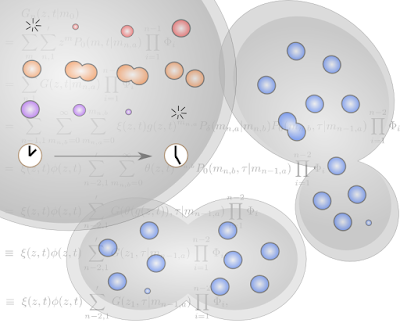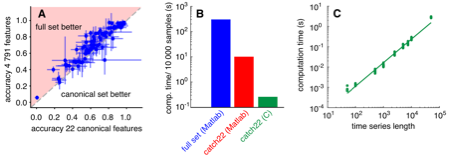## Thursday, 16 July 2015

### Generations of generating functions in dividing cells

Cell biology is a unpredictable world, as we've written about before. The important machines in our cells replicate and degrade in processes that can be described as random; and when cells divide, the partitioning of these machines between the resulting cells also looks random. The number of machines we have in our cells is important, but how can we work with numbers in this unpredictable environment?In our cells, machines are produced (red), replicate (orange), and degrade (purple) randomly with time, as well as being randomly partitioned when cells split and divide (blue). Our mathematical approach describes how the total number of machines is likely to behave and change with time and as cells divide.

Tools called "generating functions" are useful in this situation. A generating function is a mathematical function (like G(z) = z2, but generally more complicated) that encodes all the information about a random system. To find the generating function for a particular system, one needs to consider all the random things that can happen to change the state of that system, write them down in an equation (the "master equation") describing them all together, then use a mathematical trick to push that equation into a different mathematical space, where it is easier to solve. If that "transformed" equation can be solved, the result is the generating function, from which we can then get all the information we could want about a random system: the behaviour of its mean and variance, the probability of making any observation at any time, and so on.

We've gone through this mathematical process for a set of systems where individual cellular machines can be produced, replicated, and degraded randomly, and split at cell divisions in a variety of different ways. The generating functions we obtain allow us to follow this random cellular behaviour in new detail. We can make probabilistic statements about any aspect of the system at any time and after any number of cell divisions, instead of relying on assumptions that the system has somehow reached an equilibrium, or restricting ourselves to a single or small number of divisions. We've applied this tool to questions about the random dynamics of mitochondrial DNA (which we're very interested in! And this work connects explicitly with our recent eLife paper - blog article here) in cells that divide (like our cells) or "bud" (like yeast cells), but the approach is very general and we hope it will allow progress in many more biological situations. You can read about this, free, here under the title "Closed-form stochastic solutions for non-equilibrium dynamics and inheritance of cellular components over many cell divisions" in the Proceedings of the Royal Society A. Iain and Nick

### catch22 features of signals

By taking repeated measurements over time we can study the dynamics of our environment – be it the mean temperature of the UK by month, th...